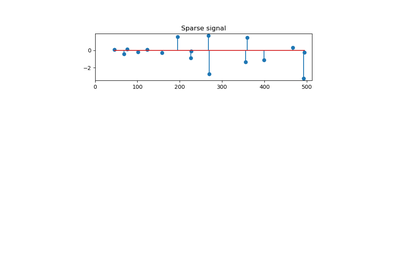# `sklearn.linear_model`.OrthogonalMatchingPursuit¶

class `sklearn.linear_model.``OrthogonalMatchingPursuit`(n_nonzero_coefs=None, tol=None, fit_intercept=True, normalize=True, precompute=’auto’)[source]

Orthogonal Matching Pursuit model (OMP)

Read more in the User Guide.

Parameters: n_nonzero_coefs : int, optional Desired number of non-zero entries in the solution. If None (by default) this value is set to 10% of n_features. tol : float, optional Maximum norm of the residual. If not None, overrides n_nonzero_coefs. fit_intercept : boolean, optional whether to calculate the intercept for this model. If set to false, no intercept will be used in calculations (e.g. data is expected to be already centered). normalize : boolean, optional, default True This parameter is ignored when `fit_intercept` is set to False. If True, the regressors X will be normalized before regression by subtracting the mean and dividing by the l2-norm. If you wish to standardize, please use `sklearn.preprocessing.StandardScaler` before calling `fit` on an estimator with `normalize=False`. precompute : {True, False, ‘auto’}, default ‘auto’ Whether to use a precomputed Gram and Xy matrix to speed up calculations. Improves performance when n_targets or n_samples is very large. Note that if you already have such matrices, you can pass them directly to the fit method. coef_ : array, shape (n_features,) or (n_targets, n_features) parameter vector (w in the formula) intercept_ : float or array, shape (n_targets,) independent term in decision function. n_iter_ : int or array-like Number of active features across every target.

Notes

Orthogonal matching pursuit was introduced in G. Mallat, Z. Zhang, Matching pursuits with time-frequency dictionaries, IEEE Transactions on Signal Processing, Vol. 41, No. 12. (December 1993), pp. 3397-3415. (http://blanche.polytechnique.fr/~mallat/papiers/MallatPursuit93.pdf)

This implementation is based on Rubinstein, R., Zibulevsky, M. and Elad, M., Efficient Implementation of the K-SVD Algorithm using Batch Orthogonal Matching Pursuit Technical Report - CS Technion, April 2008. http://www.cs.technion.ac.il/~ronrubin/Publications/KSVD-OMP-v2.pdf

Methods

 `fit`(X, y) Fit the model using X, y as training data. `get_params`([deep]) Get parameters for this estimator. `predict`(X) Predict using the linear model `score`(X, y[, sample_weight]) Returns the coefficient of determination R^2 of the prediction. `set_params`(**params) Set the parameters of this estimator.
`__init__`(n_nonzero_coefs=None, tol=None, fit_intercept=True, normalize=True, precompute=’auto’)[source]
`fit`(X, y)[source]

Fit the model using X, y as training data.

Parameters: X : array-like, shape (n_samples, n_features) Training data. y : array-like, shape (n_samples,) or (n_samples, n_targets) Target values. Will be cast to X’s dtype if necessary self : object returns an instance of self.
`get_params`(deep=True)[source]

Get parameters for this estimator.

Parameters: deep : boolean, optional If True, will return the parameters for this estimator and contained subobjects that are estimators. params : mapping of string to any Parameter names mapped to their values.
`predict`(X)[source]

Predict using the linear model

Parameters: X : {array-like, sparse matrix}, shape = (n_samples, n_features) Samples. C : array, shape = (n_samples,) Returns predicted values.
`score`(X, y, sample_weight=None)[source]

Returns the coefficient of determination R^2 of the prediction.

The coefficient R^2 is defined as (1 - u/v), where u is the residual sum of squares ((y_true - y_pred) ** 2).sum() and v is the total sum of squares ((y_true - y_true.mean()) ** 2).sum(). The best possible score is 1.0 and it can be negative (because the model can be arbitrarily worse). A constant model that always predicts the expected value of y, disregarding the input features, would get a R^2 score of 0.0.

Parameters: X : array-like, shape = (n_samples, n_features) Test samples. y : array-like, shape = (n_samples) or (n_samples, n_outputs) True values for X. sample_weight : array-like, shape = [n_samples], optional Sample weights. score : float R^2 of self.predict(X) wrt. y.
`set_params`(**params)[source]

Set the parameters of this estimator.

The method works on simple estimators as well as on nested objects (such as pipelines). The latter have parameters of the form `<component>__<parameter>` so that it’s possible to update each component of a nested object.

Returns: self :

## Examples using `sklearn.linear_model.OrthogonalMatchingPursuit`¶Orthogonal Matching Pursuit